## UNITS ...

 3rd Grade 4th Grade Unit 1 .- Measurement Unit 1 .- Measurement Unit 2 .- Time Unit 2 .- Time Unit 3 .- Money Unit 3 .- Money Unit 4 .- Geometry Unit 4 .- Geometry
 5th Grade 6th Grade Unit 1 .- Measurement Unit 1 .- Measurement Unit 2 .- Time Unit 2 .- Time Unit 3 .- Money Unit 3 .- Money Unit 4 .- Geometry Unit 4 .- Geometry

From 3rd till 6th of Primary

• 40% Activitats Tornem Enrere (Activitats sobre els temes realitzats a l’aula)

• 40% Resultats Exàmens

• 10% Fulls d’Activitats, Ampliació i/o Reforç (Activitats realitzades a casa amb o sense ajuda)

• 10% Respecte / Actitud / Participació envers l’assignatura (Quadern - Deures).

Els 7 manaments d’una bona activitat de matemàtiques!

1.- Raonar, pensar què me demanen i quin serà el procediment a seguir (+,-,X, : )

2.- Realitzar les operacions i NO esborrar-les.

3.- Realitzar SEMPRE la graella de mesura i NO esborrar-la.

4.- Escriure de què s’està xerrant (m, l, avions...)

5.- No córrer, tenim temps per a la correcta realització de les activitats i MÉS si ho feim a casa.

6.- Bona presentació i col·locació dels números.

7.- Acabar l’activitat.

## The Basic Operations

In basic mathematics there are many ways of saying the same thing:

Symbol
Words Used
+
Subtraction, Subtract, Minus, Less, Difference, Decrease, Take Away, Deduct
×
Multiplication, Multiply, Product, By, Times, Lots Of
÷
Division, Divide, Quotient, Goes Into, How Many Times

... bringing two or more numbers (or things) together to make a new total.## Subtraction is ...

... taking one number away from another.Minuend − Subtrahend = Difference

Minuend: The number that is to be subtracted from.
Subtrahend: The number that is to be subtracted.
Difference: The result of subtracting one number from another.

## Multiplication is ...

... (in its simplest form) repeated addition.

Here we see that 6+6+6 (three 6s) make 18: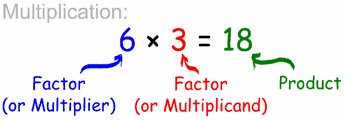It can also be said that 3+3+3+3+3+3 (six 3s) make 18

But we can also multiply by fractions or decimals, which goes beyond the simple idea of repeated addition:

Example: 3,5 × 5 = 17,5

which is 3,5 lots of 5, or 5 lots of 3,5

## Division is ...

... splitting into equal parts or groups. It is the result of "fair sharing".

Division has its own special words to remember.

Let's take the simple question of dividing 22 by 5. The answer is 4, with 2 left over. Here we see the important words: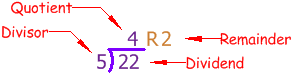Which is the same as: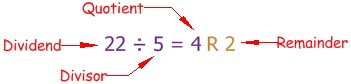## A Fraction is ...

... part of a whole.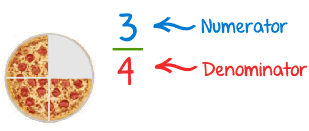A fraction is written with the bottom part (the denominator) telling us how many parts the whole is divided into,

and the top part (the numerator) telling us how many parts we have.

See Fractions for more details.

## A Decimal Number is ...

... a number that contains a Decimal Point.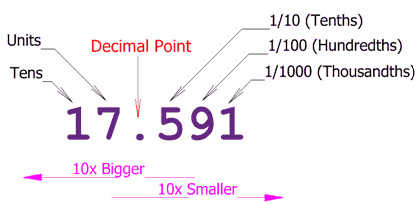See Decimals for more details.

## A Percentage is ...

... parts per 100. The symbol is %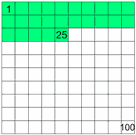Example: 25% means 25 per 100 (25% of this box is green)

See Percentages for more details.

## Average (Mean) is ...

... the sum divided by the count.

We calculate the average by adding up all the values, then divide by how many values.

### Example: What is the average of 9, 2, 12 and 5?

Add up all the values: 9 + 2 + 12 + 5 = 28

Divide by how many values (there are four of them): 28 ÷ 4 = 7

So the average is 7

# IXL Awards

## Knowledge isn't the only treasure on IXL...

In IXL, each grade level has its own themed game board, filled with awards and prizes, so practising maths is like one big treasure hunt. But you only win when you succeed!

Every time you achieve one of your maths goals—whether it's mastering a skill or practising for a certain amount of time—you just might have a surprise waiting for you. In grade 1's jungle game board, you might unlock a wild animal, or in the kindergarten toyland game board, you could stumble upon… wait, we're not telling. Keep practising, and see how many prizes you can find!

## Reaching goals the fun way

IXL is not just about helping your child learn maths—it's also about helping your child learn to love the subject. Unlike traditional workbooks and exercises, IXL's practice game boards engage students in their maths lessons. As they work for hours to uncover new prizes on their game board, they'll be gaining new maths skills and confidence along the way.